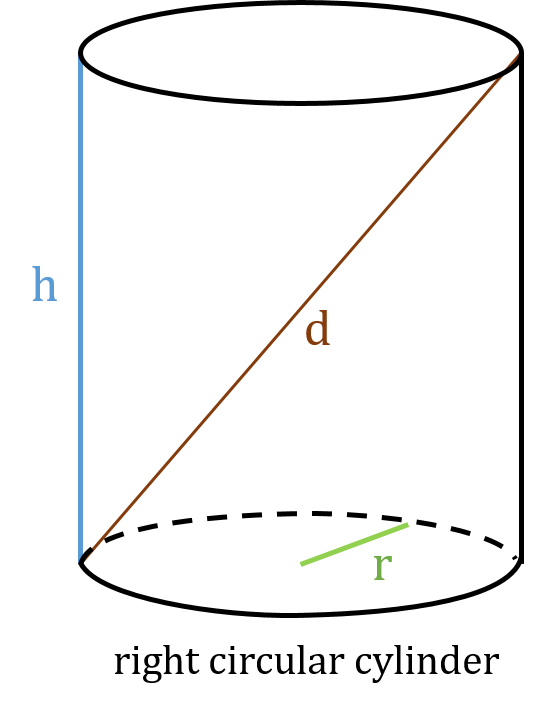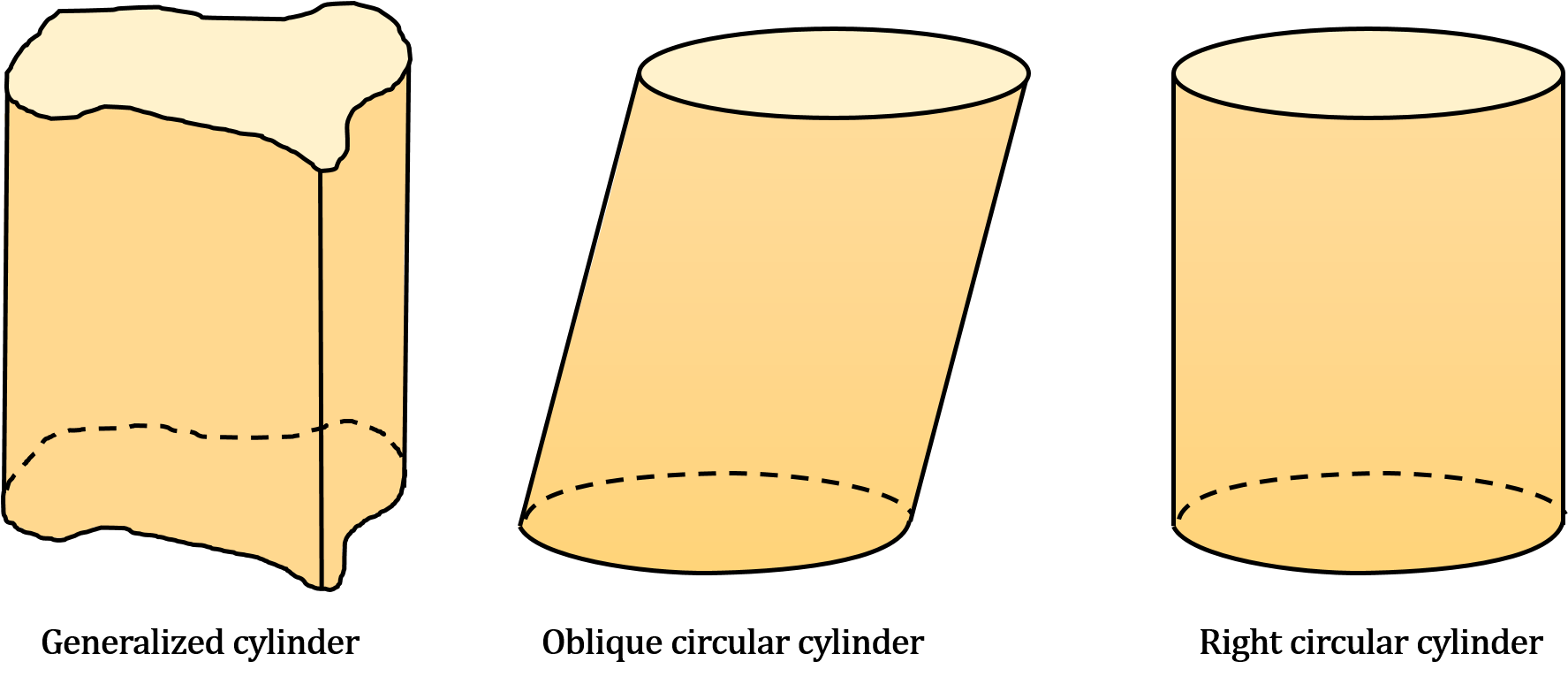# Radius of a Cylinder Calculator

Created by Dominik Czernia, PhD candidate
Reviewed by Bogna Szyk
Last updated: Sep 03, 2022

This radius of a cylinder calculator quickly finds the radius of a right circular cylinder with eight different equations. Do you need to solve a specific mathematical problem but you don't know how to find the radius of a cylinder? Try to enter a couple of parameters and see how simply this calculator works.

There are many different ways to estimate the radius of a cylinder. In the following text, we have presented eight of the radius of cylinder formulas that are used by our calculator. In most cases, you will need only two of the below quantities:• height,
• volume,
• longest diagonal,
• base surface area,
• lateral surface area,
• total surface area,
• or surface-to-volume ratio.

This radius of a cylinder calculator is a handy tool that can compute the parameters of right circular cylinders. By the radius of a cylinder we mean the radius of two congruent circles that are bases of a cylinder. That's why this type of cylinders is called circular.

You should remember that the word cylinder may correspond to different shapes (generalized cylinder). However, we usually have in mind the right circular cylinder. The cylinder is right when one of the bases lies precisely above the other. Otherwise, the cylinder is oblique.

The calculator on this site was prepared to answer the question how to find the radius of a cylinder. In our other right circular cylinder calculator, you can find more general information about cylinders. Be sure to check it out!## What's the radius of a cylinder formula?

To describe the properties of a cylinder, we usually use radius r and height h. Below, we have listed six basic equations that can be used to derive the explicit formulas of the radius of a cylinder:

1. Volume of a cylinder: V = π * r² * h,
2. Base surface area of a cylinder: A_b = 2 * π * r²,
3. Lateral surface area of a cylinder: A_l = 2 * π * r * h,
4. Total surface area of a cylinder: A = A_b + A_l,
5. Longest diagonal of a cylinder: d² = 4 * r² + h²,
6. Surface-area-to-volume ratio of a cylinder: SA:V = A / V = 2 * (r + h) / (r * h)

A radius of a cylinder is not always easy to estimate. However, with our radius of a cylinder calculator, you can now quickly compute it with the following eight radius of a cylinder formulas. They were all derived directly from the above equations.

1. Given height and volume: r = √(V / (π * h)),
2. Given height and lateral area: r = A_l / (2 * π * h),
3. Given height and total area: r = (√(h² + 2 * A / π) - h) / 2,
4. Given height and diagonal: r = √(h² + d²) / 2,
5. Given height and surface-area-to-volume ratio: r = 2 * h / (h * SA:V - 2),
6. Given volume and lateral area: r = 2 * V / A_l,
7. Given base area: r = √(A_b / (2 * π)),
8. Given lateral area and total area: r = √((A - A_l) / (2 * π)).

Do you need to calculate another parameter of a cylinder? You certainly need to check out our surface area of a cylinder calculator and cylinder height calculator too!

## How to find the radius of a cylinder?

The radius of a cylinder calculator is a useful tool prepared to solve a wide range of different problems. Sometimes you will know what is the height and the volume of a cylinder, and you won't know the radius. Another time you will have to face a more complicated problem. For example, you will know what is the height of a cylinder and the surface-area-to-volume ratio. If you ever will have to solve that kind of task, remember to use this radius of a cylinder calculator. You need to perform only basic three steps:

1. Determine which parameters of a cylinder you know.
2. Enter values of the selected quantities.
3. Read out the result of the calculations.

Isn't that simple? Moreover, with our calculator, you can choose units of every parameter you want. It can be very helpful in many calculations! If you want to learn more about unit conversions, be sure to check out the length and the volume conversion tools as well!

## FAQ

### How do I find the radius of a cylinder given its volume?

To determine the radius of a cylinder from its volume, you also need to know the height (altitude) of this cylinder:

1. Multiply the height by π ≈ 3.14.
2. Divide the volume by the result from Step 1.
3. Take the square root of the result from Step 2.
4. You've got the radius! It wasn't that hard, was it?

### How do you find the radius given the diameter?

To determine the radius of a circle (e.g., the cylinder base), you need to divide the diameter by 2. For instance, if the diameter is 7, then the radius is 3.5.

### What is the radius of a cylinder?

A cylinder is a solid that has two identical circular bases. The radius of a cylinder is the radius of the base of this cylinder.

Dominik Czernia, PhD candidateHeight (h)
in
Volume
cu in
Diagonal (d)
in
Surface to volume ratio
1/
in
Surface areas
Base surface area
in²
Lateral surface area
in²
Total surface area
in²
in
People also viewed…

### Flat vs. round Earth

Omni's not-flat Earth calculator helps you perform three experiments that prove the world is round.

### Free fall

Our free fall calculator can find the velocity of a falling object and the height it drops from.

### Rational zeros

The rational zeros calculator lists all possible rational zeros of any given integer-coefficient polynomial, and pick those that are actual rational zeros of the polynomial.

### Unit circle

Learn about the trigonometric functions with this unit circle calculator.